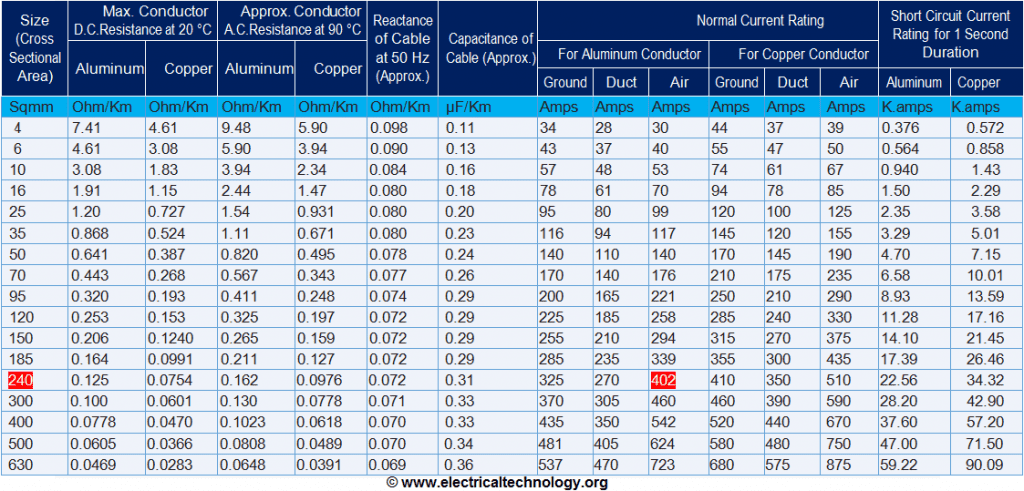# LT Motor:

The low voltage or low tension motor is called LT motor. the LT motors mostly operated between the voltage ranges from 240v to 415v .

LT motors mostly having high current and low voltage. moreover we can say up to 1KV motors are LT motors.

## HT motor:

The high voltage or high tension motor is called HT motor. the HT motors mostly operated between the voltages range from 3.3KV to 11KV. HT motors mostly having low current and high voltages. moreover we can say above 1KV motors are HT motors.

### How to calculate cable size of LT motor:

Now we are going to calculate cable size of LT motor with examples, here we will consider 100kw LT motors and motor having efficiency 94%, power factor 0.8 , system terminal voltage 415v and cable length is 200m. so we will find cable size for this LT motor.

Cable Size Calculation of 100 KW LT Motor

generally we know power formula P= 3 x V x I CosΦ

let I = P / 3 V x  CosΦ

we know 3= 1.732, CosΦ = power factor = 0.8

and voltage v=415v so apply these values into above equation

= 100000/(1.732x415x0.8×0.94)

~ 185 A

This is full load current for LT motor in normal condition. but in real condition we need to consider some other factors to size the cable.

let us consider normal ambient temperature is 40* C. in case the ambient temperature is increase then current carrying  capacity of the cable is de rated. that could be understand by below de rating table

Suppose we are run the cable in Air laid on cable tray,

 Air Temperature in Deg. 20° 25° 30° 35° 40° 45° 50° 55° Normal PVC 1.32 1.25 1.16 1.09 1.00 0.90 0.80 0.80 De-Rating factors HR PVC 1.22 1.17 1.12 1.06 1.00 0.94 0.87 0.80 XLPE 1.20 1.16 1.11 1.06 1.00 0.95 0.88 0.82

Rating factors related to variation in ambient air temperature

Normally cable de rating factor formula is

= K1*K2

K1= Temperature Correction Factor

K2= Cable Grouping Factor

Temperature Correction Factor, K1 when cable is in the Air = 0.88 (for 50* Amb temp & XLPE cable)

Grouping factor of the cable also de rate the current carrying capacity of the cable. if more than 3 cables run together in a single tray. then it will be produce heat it self, and this will increase the temperature of the cable. then current carrying capacity of the cable is de rated.

lets consider some worst cases , i.e. 3 trays parallel to each other having 9 cables each touching to each other.No. of racks No. of cables per rack No. of cables per rack 1 2 3 6 9 1 2 3 6 9 1 1.00 0.98 0.96 0.93 0.92 1.00 0.84 0.80 0.75 0.73 2 1.00 0.95 0.93 0.90 0.89 1.00 0.80 0.76 0.71 0.69 3 1.00 0.94 0.92 0.89 0.88 1.00 0.78 0.74 0.70 0.68 6 1.00 0.93 0.90 0.87 0.86 1.00 0.76 0.72 0.65 0.66

Cable Grouping Factor (No of Tray Factor), K2 = 0.68 (for 3 trays having 9 cable each)

Total de-rating factor = K1 x K2

=  0.88×0.68 = 0.5984

Let’s consider 3 core, 185 Sq.mm, Aluminum, XLPE, Armored cable for single run

Click to enlarge the tablelet see that details for    3 Core, Aluminum/Copper Conductor, XLPE Insulated, Armored Cables

Current capacity of 185 Sq.mm XLPE Armored aluminum cable in Air is 339 Amp

Total de-rating current of 185 Sq.mm Cable = 339×0.5984 = 202.85 Amp

Resistance = 0.211 Ω/Km and
Reactance = 0.072 Ω /Km

•
 Estimated Voltage Drops in PVC/XLPE Aluminum Cables For A.C. System (Voltage drop – Volts/Km/Amps) Nominal area of conductor (sq. mm) P.V.C. Cable XLPE Cable Single Phase Three Phase SinglePhase Three System 1.5 43.44 37.62 46.34 40.13 2.5 29.04 25.15 30.98 26.83 4 17.78 15.40 18.98 16.44 6 11.06 9.58 11.80 10.22 10 7.40 6.41 7.88 6.82 16 4.58 3.97 4.90 4.24 25 2.89 2.50 3.08 2.67 35 2.10 1.80 2.23 1.94 50 1.55 1.30 1.65 1.44 70 1.10 0.94 1.15 1.00 95 0.79 0.68 0.83 0.70 120 0.63 0.55 0.66 0.56 150 0.52 0.46 0.55 0.48 185 0.42 0.37 0.44 0.40 240 0.34 0.30 0.35 0.30 300 0.28 0.26 0.30 0.26 400 0.24 0.22 0.24 0.22 500 0.23 0.20 0.23 0.20 630 0.20 0.18 0.21 0.18 800 0.19 – 0.20 – 1000 0.18 – 0.18 –

from above table we consider Voltage drop, for 3 phase 185sqmm XLPE cable is 0.40

voltage drop V2 = 0.4 Volts/Km/Amp

= 0.4x217x200/1000

= 17.3 V

Terminal voltage at Motor, V2 = 415-13 = 402 V

% Drop = (V2 – V1)/(V1)

= (415 – 402)x100/(415)

= 4.18%

To decide 185 Sq.mm cable, cable selection  should be satisfied following condition

1. Cable derating Amp (202.85 Amp)is higher than full load current of load (185 Amp) = OK
2. Cable voltage Drop (4.18%)is less than defined voltage drop (10%) = OK
3. Cable short circuit capacity (17.39 KA) is higher than system short circuit capacity at that point ( X KA) = OK

So 100kw LT motor for cable 200m length required 185sqmm XLPE cable.